Hostname: page-component-797576ffbb-tx785 Total loading time: 0 Render date: 2023-12-06T08:08:58.846Z Has data issue: false Feature Flags: { "corePageComponentGetUserInfoFromSharedSession": true, "coreDisableEcommerce": false, "useRatesEcommerce": true } hasContentIssue false

# A NOTE ON THE DIOPHANTINE EQUATION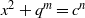${x}^{2} + {q}^{m} = {c}^{n}$

Published online by Cambridge University Press:  26 November 2013

## Abstract

Core share and HTML view are not possible as this article does not have html content. However, as you have access to this content, a full PDF is available via the ‘Save PDF’ action button.

Let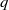$q$ be an odd prime such that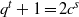${q}^{t} + 1= 2{c}^{s}$, where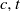$c, t$ are positive integers and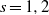$s= 1, 2$. We show that the Diophantine equation${x}^{2} + {q}^{m} = {c}^{n}$ has only the positive integer solution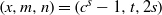$(x, m, n)= ({c}^{s} - 1, t, 2s)$ under some conditions. The proof is based on elementary methods and a result concerning the Diophantine equation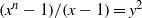$({x}^{n} - 1)/ (x- 1)= {y}^{2}$ due to Ljunggren. We also verify that when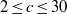$2\leq c\leq 30$ with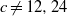$c\not = 12, 24$, the Diophantine equation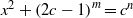${x}^{2} + \mathop{(2c- 1)}\nolimits ^{m} = {c}^{n}$ has only the positive integer solution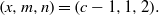$(x, m, n)= (c- 1, 1, 2).$

Type
Research Article
Information

## References

Arif, S. A. and Abu Muriefah, F. S., ‘On the Diophantine equation${x}^{2} + {q}^{2k+ 1} = {y}^{n}$’, J. Number Theory 95 (2002), 95100.Google Scholar
Cao, Z. and Dong, X., ‘On Terai’s conjecture’, Proc. Japan Acad. 74A (1998), 127129.Google Scholar
Cenberci, S. and Senay, H., ‘The Diophantine equation${x}^{2} + {B}^{m} = {y}^{n}$’, Int. J. Algebra 3 (2009), 657662.Google Scholar
Cenberci, S. and Senay, H., ‘The Diophantine equation${x}^{2} + {q}^{m} = {p}^{n}$’, Int. J. Contemp. Math. Sci. 4 (2009), 11811191.Google Scholar
Chen, X. and Le, M., ‘A note on Terai’s conjecture concerning Pythagorean numbers’, Proc. Japan Acad. 74A (1998), 8081.Google Scholar
Jeśmanowicz, L., ‘Some remarks on Pythagorean numbers’, Wiad. Mat. 1 (1955/1956), 196202 (in Polish).Google Scholar
Le, M., ‘A Note on the Diophantine equation${x}^{2} + {b}^{y} = {c}^{z}$’, Acta Arith. 71 (1995), 253257.Google Scholar
Le, M., ‘On Terai’s conjecture concerning Pythagorean numbers’, Acta Arith. 100 (2001), 4145.Google Scholar
Ljunggren, W., ‘Some theorems on indeterminate equations of the form$\frac{{x}^{n} - 1}{x- 1} = {y}^{q}$’, Norsk Mat. Tidsskr. 25 (1943), 1720 (in Norwegian).Google Scholar
Sierpiński, W., ‘On the equation${3}^{x} + {4}^{y} = {5}^{z}$’, Wiadom. Mat. 1 (1955/1956), 194195 (in Polish).Google Scholar
Terai, N., ‘The Diophantine quation${x}^{2} + {q}^{m} = {p}^{n}$’, Acta Arith. 63 (1993), 351358.Google Scholar
Yuan, P. and Wang, J., ‘On the Diophantine equation${x}^{2} + {b}^{y} = {c}^{z}$’, Acta Arith. 84 (1998), 145147.Google Scholar
Zhu, H. L., ‘A note on the Diophantine equation${x}^{2} + {q}^{m} = {y}^{3}$’, Acta Arith. 146 (2011), 195202.Google Scholar